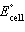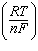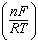Name:    Chapter 20 Online Practice

Multiple Choice
Identify the letter of the choice that best completes the statement or answers the question.

1.

In the following reaction,

2 Fe3+(aq) + Zn(s) ® 2 Fe2+(aq) + Zn2+(aq)
 a. Fe3+(aq) is the reducing agent and Zn(s) is the oxidizing agent. b. Zn(s) is the reducing agent and Fe3+(aq) is the oxidizing agent. c. Fe3+(aq) is the reducing agent and Fe2+(aq) is the oxidizing agent. d. Zn(s) is the reducing agent and Zn2+(aq) is the oxidizing agent. e. Zn(s) is the reducing agent and Fe2+(aq) is the oxidizing agent.

2.

Assuming the following reaction proceeds in the forward direction,

Ni2+(aq) + Cr(s) ® Ni(s) + Cr2+(aq)
 a. Ni2+(aq) is oxidized and Cr(s) is reduced. b. Ni2+(aq) is oxidized and Ni(s) is reduced. c. Ni(s) is oxidized and Ni2+(aq) is reduced. d. Cr(s) is oxidized and Cr2+(aq) is reduced. e. Cr(s) is oxidized and Ni2+(aq) is reduced.

3.

The following reaction occurs spontaneously.

2 H+(aq) + Ca(s) ® Ca2+(aq) + H2(g)

Write the balanced reduction half-reaction.
 a. 2 H+(aq) + 2 e- ® H2(g) b. 2 H+(aq) ® H2(g) + 2 e- c. H2(g) ® 2 H+(aq) + 2 e- d. Ca(s) + 2 e- ® Ca2+(aq) e. Ca(s) ® Ca2+(aq) + 2 e-

4.

Write a balanced half-reaction for the reduction of ClO3-(aq) to Cl2(g) in an acidic solution.
 a. 2 ClO3-(aq) + 6 H+(aq) + 10 e- ® Cl2(g) + 6 OH-(aq) b. 2 ClO3-(aq) + 12 H+(aq) + 5 e- ® Cl2(g) + 6 H2O(l) c. 2 ClO3-(aq) + 10 e- ® Cl2(g) + 6 H2O(l) + 3 O2(g) d. 2 ClO3-(aq) + 12 H+(aq) + 10 e- ® Cl2(g) + 6 H2O(l) e. 2 ClO3-(aq) + 18 H+(aq) ® Cl2(g) + 6 H3O+(aq)

5.

Write a balanced chemical equation for the following reaction in an acidic solution.

Cr2O72-(aq) + Br-(aq) ® Cr3+(aq) + Br2(aq)
 a. Cr2O72-(aq) + 2 Br-(aq) ® 2 Cr3+(aq) + Br2(aq) b. Cr2O72-(aq) + 6 Br-(aq) + 14 H+(aq) ® 3 Br2(aq) + 2 Cr3+(aq) + 7 H2O(l) c. Cr2O72-(aq) + 2 Br-(aq) + 14 H+(aq) ® 2 Cr3+(aq) + Br2(aq) + 7 H2O(l) d. Cr2O72-(aq) + 2 Br-(aq) + 7 H+(aq) ® 2 Cr3+(aq) + Br2(aq) + 7 OH-(aq) e. Cr2O72-(aq) + 6 Br-(aq) + 7 H+(aq) ® 2 Cr3+(aq) + 3 Br2(aq) + 7 OH-(aq)

6.

All of the following statements concerning voltaic cells are true EXCEPT
 a. a salt bridge allows cations and anions to move between the half-cells. b. electrons flow from the cathode to the anode. c. reduction occurs at the cathode. d. a voltaic cell can be used as a source of energy. e. a voltaic cell consists of two-half cells.

7.

What is the correct cell notation for a voltaic cell based on the reaction below?

Ni2+(aq) + Zn(s) ® Ni(s) + Zn2+(aq)
 a. Zn(s) ï Zn2+(aq)Ni2+(aq) ï Ni(s) b. Zn(s)Zn2+(aq), Ni2+(aq) ï Ni(s) c. Ni(s)Ni2+(aq), Zn2+(aq)Zn(s) d. Ni(s) ï Zn2+(aq)Ni2+(aq) ï Zn(s) e. Ni(s) ï Ni2+(aq)Zn2+(aq) ï Zn(s)

8.

Which of the following half-reactions has been assigned a standard reduction potential, E°, of 0.00 Volts?
 a. 2 H2O(l) + 2 e- ® H2(g) + 2 OH-(aq) b. O2(g) + 4 e- ® 2 O2-(aq) c. Hg2Cl2(s) + 2 e- ® 2 Hg(l) + 2 Cl-(aq) d. Li+(aq) + e- ® Li(s) e. 2 H+(aq) + 2 e- ® H2(g)

9.

Use the standard reduction potentials below to determine which element or ion is the best oxidizing agent.

Br2(l) + 2 e- ® 2 Br-(aq) E° = +1.08 V
Hg2+(aq) + e- ® Hg(l) E° = +0.86 V
Ni2+(aq) + 2 e- ® Ni(s) E° = -0.25 V
 a. Br2 b. Br- c. Hg2+ d. Ni2+ e. Ni

10.

Which of the following species are likely to behave as oxidizing agents: K+, MnO4-, Cr2O72-, and I-?
 a. K+ only b. MnO4- and Cr2O72- c. MnO4-, Cr2O72-, and I- d. Cr2O72- and I- e. I- only

11.

Consider the following half-reactions:

F2(g) + 2 e- ® 2 F-(aq) E° = +2.87 V
Cu2+(aq) + 2 e- ® Cu(s) E° = +0.34 V
Sn2+(aq) + 2 e- ® Sn(s) E° = -0.14 V
Al3+(aq) + 3 e- ® Al(s) E° = -1.66 V
Na+(aq) + e- ® Na(s) E° = -2.71 V

Which of the above elements or ions will oxidize Sn(s)?
 a. Cu(s) and Al3+(aq) b. F-(aq) and Cu(s) c. F2(g) and Cu2+(aq) d. Al(s) and Na(s) e. Al3+(aq) and Na+(aq)

12.

Given the following two half-reactions, determine which overall reaction is spontaneous and calculate its standard cell potential.

Cd2+(aq) + 2 e- ® Cd(s) E° = -1.21 V
Sn2+(aq) + 2 e- ® Sn(s) E° = -0.14 V
 a. Cd2+(aq) + Sn(s) ® Cd(s) + Sn2+(aq)= +1.07 V b. Cd2+(aq) + Sn(s) ® Cd(s) + Sn2+(aq)= -1.35 V c. Cd(s) + Sn2+(aq) ® Cd2+(aq) + Sn(s)= +1.07 V d. Cd(s) + Sn2+(aq) ® Cd2+(aq) + Sn(s)= -1.07 V e. Cd(s) + Sn2+(aq) ® Cd2+(aq) + Sn(s)= +1.35 V

13.

Calculatefor the reaction below,

Tl+(aq) + 2 Cl-(aq) ® 2 Tl(s) + Cl2(g)

given the following standard reduction potentials.

Cl2(g) + 2 e- ® 2 Cl-(aq) E° = +1.36 V
Tl+(aq) + e- ® Tl(s) E° = -0.34 V
 a. -2.04 V b. -1.70 V c. 1.02 V d. +1.70 V e. +2.04 V

14.

Which of the following equations is a correct form of the Nernst equation?
 a. E = E° -ln Q b. E = E° + logc. E = E° -log Q d. E = E° -ln Q e. E = E° -ln Q

15.

A Faraday, F, is defined as
 a. the charge on a single electron. b. the charge, in coulombs, carried by one mole of electrons. c. the voltage required to reduce one mole of reactant. d. the current required to reduce one mole of reactant. e. the charge passed by one ampere of current in one second.

16.

Calculate the cell potential, at 25 °C, based upon the overall reaction

3 Cu2+(aq) + 2 Al(s) ® 3 Cu(s) + 2 Al3+(aq)

if [Cu2+] = 0.75 M and [Al3+] = 0.0010 M. The standard reduction potentials are as follows:

Cu2+(aq) + e- ® Cu(s) E° = +0.34 V
Al3+(aq) + 3 e- ® Al(s) E° = -1.66 V
 a. -2.11 V b. -1.26 V c. +1.94 V d. +2.06 V e. +2.11 V

17.for the following galvanic cell is +0.254 V.

Hg22+(aq) + 2 I-(aq) ® 2 Hg(l) + I2(s)

What is DG° for this reaction?
 a. -49.0 kJ b. -24.5 kJ c. +24.5 kJ d. +49.0 kJ e. +197 kJ

18.

Calculate the equilibrium constant for the following reaction at 25 °C,

Co2+(aq) + 2 Cr2+(aq) ® Co(s) + 2 Cr3+(aq)

given the following thermodynamic information.

Co2+(aq) + 2 e- ® Co(s) E° = -0.28 V
Cr3+(aq) + e- ® Cr2+(aq) E° = -0.41 V
 a. 5 ´ 10-24 b. 7 ´ 10-11 c. 4 ´ 10-5 d. 2 ´ 104 e. 1 ´ 1010

19.

Al3+ is reduced to Al(s) at an electrode. If a current of 2.00 ampere is passed for 48 hours, what mass of aluminum is deposited at the electrode? Assume 100% current efficiency.
 a. 3.58 g b. 32.2 g c. 48.3 g d. 96.6 g e. 2.90 ´ 102 g